### SMALL Function Examples in Excel, VBA, & Google Sheets

This tutorial demonstrates how to use the Excel SMALL Function in Excel to calculate the nth smallest value.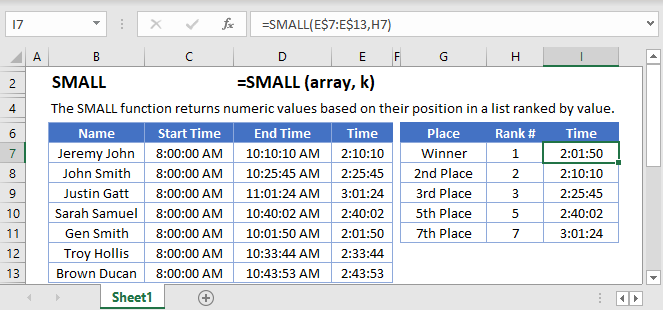## SMALL Function Overview

The SMALL Function Calculates the kth smallest value.

To use the SMALL Excel Worksheet Function, select a cell and type: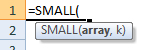(Notice how the formula inputs appear)

### SMALL function Syntax and inputs:

k – The position of the value you want to return. Example: 6 would return the 6th smallest value.

## How to use the SMALL Function

The SMALL Function returns the k-th smallest number from a data range.

To use the SMALL Function, use a formula like this: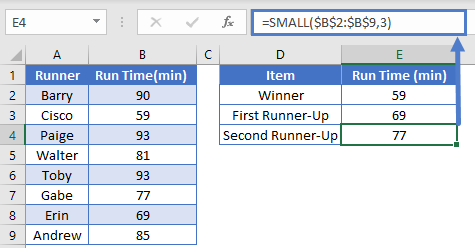Here we are searching for the 3rd smallest number (or the second runner up) in the range B2:B9.

### Out of Range Error

If you enter a number k, greater than the number of items in the range,  SMALL will return #NUM! error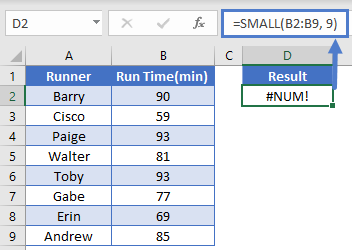Notice here we are searching for the 9th smallest number in a range of only 8 numbers. So SMALL returns #NUM!.

### Non-numeric Data

The SMALL Function will completely ignore non-numeric data, acting just as if the non-numeric data did not exist.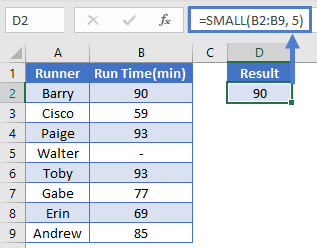## SMALL If

We can use the SMALL Function with criteria as well to perform a “small if”. For example, let’s say we want to find out who is the fastest runner over the age of 25.

We will use this array formula: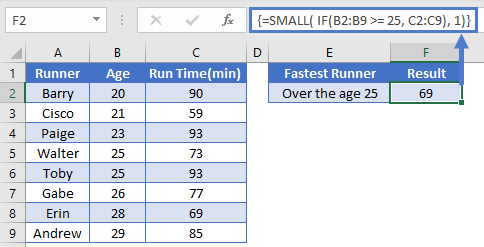Note: When building array functions, you must press CTRL + SHIFT + ENTER instead of just ENTER after creating your formula.

You’ll notice how the curly brackets appear. You can not just manually type in the curly brackets; you must use CTRL + SHIFT + ENTER.

## Sort with the SMALL Function

One very useful, but not well known, use case for the SMALL Function is to sort data in ascending (smallest to largest) order. To sort data, we combine the SMALL Function with the ROW Function.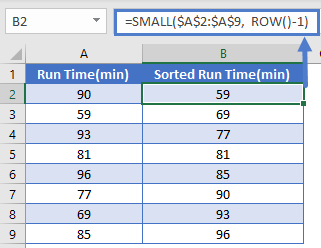This function works by using the ROW Function to define the k-value (row 2 for the smallest, row 3 second smallest, etc.). It starts with k =1 (1st smallest) and goes up to k = 8 (8th smallest number) thus sorting them from smallest to largest. To learn more read our tutorial on Sorting with the SMALL and LARGE Functions.

Note: The above formula uses absolute references (the \$ signs) to lock cell references when copying formulas. If you aren’t familiar with this, please read our Excel References Guide (link to: https://www.automateexcel.com/excel/basics/cell-references?preview=true#Absolute_Frozen_and_Relative_References  )

## SMALL function in Google Sheets

The SMALL function works exactly the same in Google Sheets as in Excel.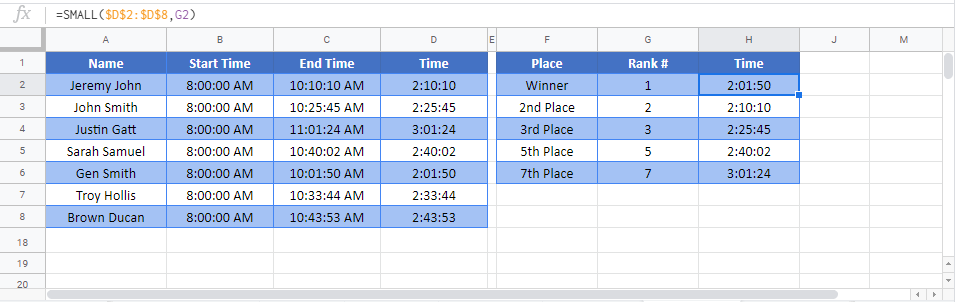## SMALL Examples in VBA

You can also use the SMALL function in VBA. Type:
`application.worksheetfunction.small(array,k)`
For the function arguments (array, etc.), you can either enter them directly into the function, or define variables to use instead.